### Home > PC > Chapter 1 > Lesson 1.2.2 > Problem1-82

1-82.
1. Some of the expressions given below are currently written in the form pq . If the expression is of the form pq , identify p and q. If not, answer “not in pq form.” Homework Help ✎

 Example: (3x)(2 + y) Solution: p = 3x, q = 2 + y Example: 5(7)2 Solution: Not in pq form (even though part of it is).
1. 62

2. 10 · 62

3. (x + 3y)(2−r)

4. 43 − 34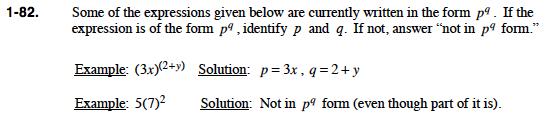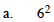p = 6
q = 2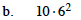not the correct form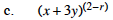p = x + 3y
q = 2 − r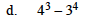not the correct form